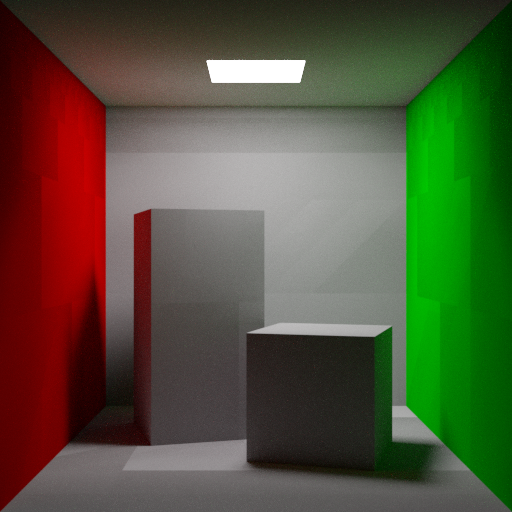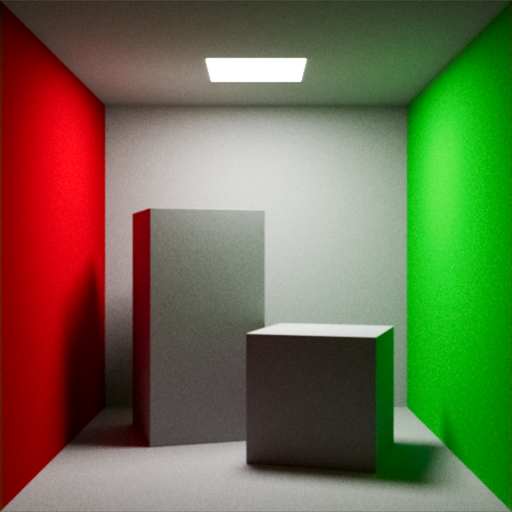# # 149 行代码的康奈尔盒子Cornell Box BlenderCornell Box Taichi
``````import taichi as ti                                                                # of course, we need taichi
from taichi.math import *                                                # need common mathematical operations

ti.init(arch=ti.gpu, default_ip=ti.i32, default_fp=ti.f32)        # initialize, use GPU, set default ip and fp

image_resolution = (512, 512)                                         # resolution of the image, not too large
image_buffer = ti.Vector.field(4, float, image_resolution)    # image buffer field for recording sample counts
image_pixels = ti.Vector.field(3, float, image_resolution)           # for output display pixels to the screen

Ray = ti.types.struct(origin=vec3, direction=vec3, color=vec3)      # the struct representing camera light ray
Material = ti.types.struct(albedo=vec3, emission=vec3)             # Cornell Box need only albedo and emission
Transform = ti.types.struct(position=vec3, rotation=vec3, scale=vec3)          # Transformation of SDF objects
SDFObject = ti.types.struct(distance=float, transform=Transform, material=Material)              # SDF objects
HitRecord = ti.types.struct(object=SDFObject, position=vec3, distance=float, hit=bool)   # for ray-hit-surface

objects    = SDFObject.field(shape=8)                           # field for storing SDF objects with 8 objects
objects = SDFObject(transform=Transform(vec3(0, 0, -1), vec3(0, 0, 0), vec3(1, 1, 0.2)),
material=Material(vec3(1, 1, 1)*0.4, vec3(1)))                                        # wall 1
objects = SDFObject(transform=Transform(vec3(0, 1, 0), vec3(90, 0, 0), vec3(1, 1, 0.2)),
material=Material(vec3(1, 1, 1)*0.4, vec3(1)))                                        # wall 2
objects = SDFObject(transform=Transform(vec3(0, -1, 0), vec3(90, 0, 0), vec3(1, 1, 0.2)),
material=Material(vec3(1, 1, 1)*0.4, vec3(1)))                                        # wall 3
objects = SDFObject(transform=Transform(vec3(-1, 0, 0), vec3(0, 90, 0), vec3(1, 1, 0.2)),
material=Material(vec3(1, 0, 0)*0.5, vec3(1)))                                        # wall 4
objects = SDFObject(transform=Transform(vec3(1, 0, 0), vec3(0, 90, 0), vec3(1, 1, 0.2)),
material=Material(vec3(0, 1, 0)*0.5, vec3(1)))                                        # wall 5
objects = SDFObject(transform=Transform(vec3(-0.275, -0.3, -0.2), vec3(0, 112, 0), vec3(0.25, 0.5, 0.25)),
material=Material(vec3(1, 1, 1)*0.4, vec3(1)))                                    # taller box
objects = SDFObject(transform=Transform(vec3(0.275,-0.55, 0.2), vec3(0, -197, 0), vec3(0.25, 0.25, 0.25)),
material=Material(vec3(1, 1, 1)*0.4, vec3(1)))                                           # box
objects = SDFObject(transform=Transform(vec3(0, 0.809, 0), vec3(90, 0, 0), vec3(0.2, 0.2, 0.01)),
material=Material(vec3(1, 1, 1)*1, vec3(100)))                                         # light

@ti.func
def angle(a: vec3) -> mat3:                                          # convert Euler angles to rotation matrix
s, c = sin(a), cos(a)                                          # first calculate the two axial projections
return mat3(c.z, s.z, 0, -s.z, c.z, 0, 0, 0, 1) @ \
mat3(c.y, 0, -s.y, 0, 1, 0, s.y, 0, c.y) @ \
mat3(1, 0, 0, 0, c.x, s.x, 0, -s.x, c.x)      # convert to rotation matrix in XYZ and multiply left

@ti.func
def signed_distance(obj: SDFObject, pos: vec3) -> float:     # calc the signed distance from pos to SDF object
p = angle(radians(obj.transform.rotation)) @ (pos - obj.transform.position)    # translate and then rotate
q = abs(p) - obj.transform.scale
return length(max(q, 0)) + min(max(q.x, max(q.y, q.z)), 0)               # return the sdf value of the box

@ti.func
def nearest_object(p: vec3) -> SDFObject:                                        # find the nearest sdf object
o = objects; o.distance = abs(signed_distance(o, p))                   # we start with the first object
for i in range(1, 8):                                                                  # for all 8 objects
oi = objects[i]; oi.distance = abs(signed_distance(oi, p))     # handling the interior of SDF with abs
if oi.distance < o.distance: o = oi                  # we need the nearest object to step into the ray
return o                                             # this can also be seen as a concatenation of the SDF

@ti.func
def calc_normal(obj: SDFObject, p: vec3) -> vec3: # representing the surface normal by the gradient of the SDF
e = vec2(1, -1) * 0.5773 * 0.005                # calculation of gradients using the Tetrahedron technique
return normalize(e.xyy * signed_distance(obj, p + e.xyy) + \
e.yyx * signed_distance(obj, p + e.yyx) + \
e.yxy * signed_distance(obj, p + e.yxy) + \
e.xxx * signed_distance(obj, p + e.xxx) )

@ti.func
def raycast(ray: Ray) -> HitRecord:                 # ray marching to obtain the intersection with the surface
record = HitRecord(distance=0.0005)                                  # step a little off the surface first
for _ in range(256):                                                      # need a maximum number of steps
record.position  = ray.origin + record.distance * ray.direction
record.object    = nearest_object(record.position)    # according to the nearest distance ray marching
record.distance += record.object.distance                                             # sphere tracing
record.hit       = record.object.distance < 0.00001         # less than the surface thickness is a hit
if record.distance > 2000.0 or record.hit: break                        # no need to continue stepping
return record

@ti.func
def hemispheric_sampling(normal: vec3) -> vec3:           # choose a random direction in the normal hemisphere
z = 2.0 * ti.random() - 1.0
a = ti.random() * 2.0 * pi
xy = sqrt(1.0 - z*z) * vec2(sin(a), cos(a))
return normalize(normal + vec3(xy, z))

@ti.func
def raytrace(ray: Ray) -> Ray:                                                                  # Path Tracing
for i in range(3):                   # 3 times is already enough to bring Global Illumination to the scene
inv_pdf = exp(float(i) / 128.0)
roulette_prob = 1.0 - (1.0 / inv_pdf)  # Russian Roulette for spreading the computation between frames
if ti.random() < roulette_prob: ray.color *= roulette_prob; break                     # end of tracing

record = raycast(ray)                           # calculate the intersection of the ray with the scene
if not record.hit: ray.color = vec3(0); break                           # not hitting the light source

normal  = calc_normal(record.object, record.position)     # calc the normal of the intersection points
ray.direction = hemispheric_sampling(normal)                # approximate diffuse reflection direction
ray.color *= record.object.material.albedo                  # ray needs to be multiplied by the albedo
ray.origin = record.position                                         # update light departure position

intensity  = dot(ray.color, vec3(0.299, 0.587, 0.114))              # calculating the intensity of ray
ray.color *= record.object.material.emission                                # multiplying the emission
visible    = dot(ray.color, vec3(0.299, 0.587, 0.114))                                # new brightness
if intensity < visible or visible < 0.000001: break           # too dark or arrive at the light source
return ray

@ti.kernel
def render(camera_position: vec3, camera_lookat: vec3, camera_up: vec3):
for i, j in image_pixels:                                         # iterate through all pixels in parallel
buffer = image_buffer[i, j]                                                     # current buffer color

z = normalize(camera_position - camera_lookat)
x = normalize(cross(camera_up, z))                          # calculating the camera coordinate system
y = cross(z, x)

half_width = half_height = tan(radians(35) * 0.5)           # calculate camera frame position and size
lower_left_corner = camera_position - half_width * x - half_height * y - z
horizontal = 2.0 * half_width  * x
vertical   = 2.0 * half_height * y

uv = (vec2(i, j) + vec2(ti.random(), ti.random())) / vec2(image_resolution)             # oversampling
po = lower_left_corner + uv.x * horizontal + uv.y * vertical
rd = normalize(po - camera_position)                          # calculation of ray direction by camera

ray = raytrace(Ray(camera_position, rd, vec3(1)))                                       # Path Tracing
buffer += vec4(ray.color, 1.0)              # accumulate colors and record the number of accumulations
image_buffer[i, j] = buffer                                                      # updating the buffer

color = buffer.rgb / buffer.a                                  # calculate the average value of colors
color = pow(color, vec3(1.0 / 2.2))                     # Gamma correction, then use ACES tone mapping
color = mat3(0.597190, 0.35458, 0.04823, 0.07600, 0.90834, 0.01566, 0.02840, 0.13383, 0.83777) @ color
color = (color * (color + 0.024578) - 0.0000905) / (color * (0.983729 * color + 0.4329510) + 0.238081)
color = mat3(1.60475, -0.531, -0.0736, -0.102, 1.10813, -0.00605, -0.00327, -0.07276, 1.07602) @ color
image_pixels[i, j] = clamp(color, 0, 1)  # write pixels, clamp the brightness that cannot be displayed

def main():
window = ti.ui.Window("Cornell Box", image_resolution)                                     # create window
canvas = window.get_canvas()
while window.running:                                                            # main loop of the window
render(vec3(0, 0, 3.5), vec3(0, 0, -1), vec3(0, 1, 0))        # set the camera parameters, then render
canvas.set_image(image_pixels)                                              # writing pixels to canvas
window.show()                                                                # continue to show window

if __name__ == '__main__':
main()                                                                             # By HK-SHAO 2023/01/30
# ------------------------------------------------------------------------------------------------------------
``````

sorry...

• 抱歉我成了“标题党”，其实 139 行就够了